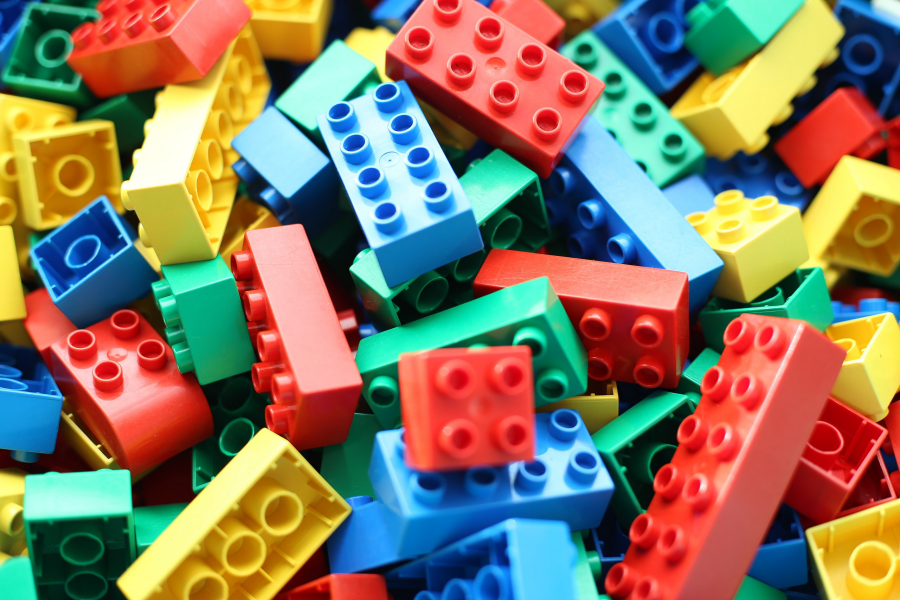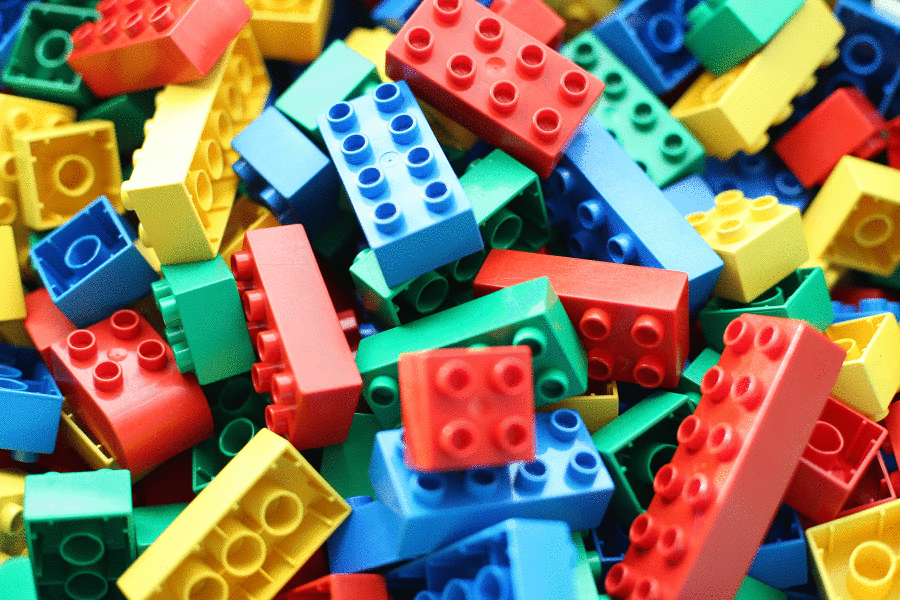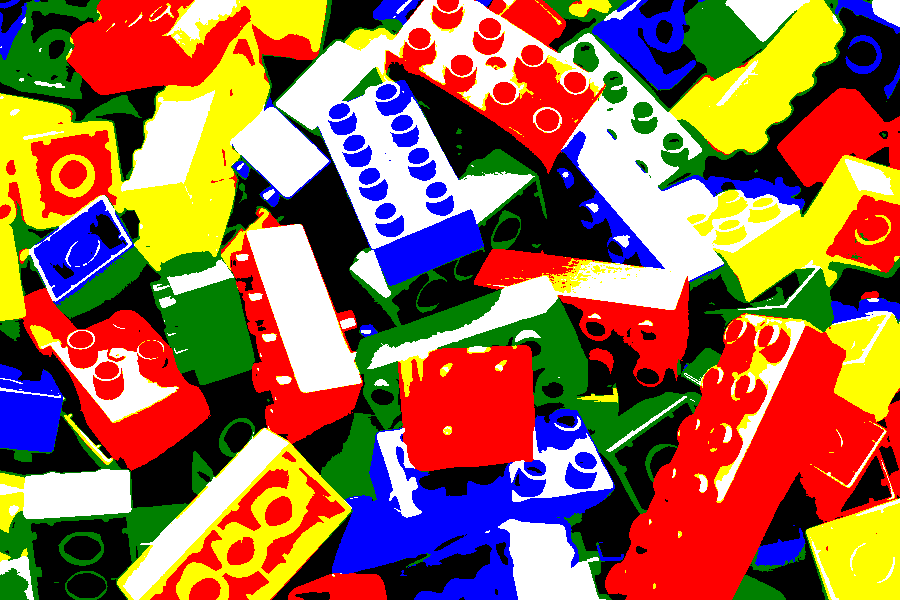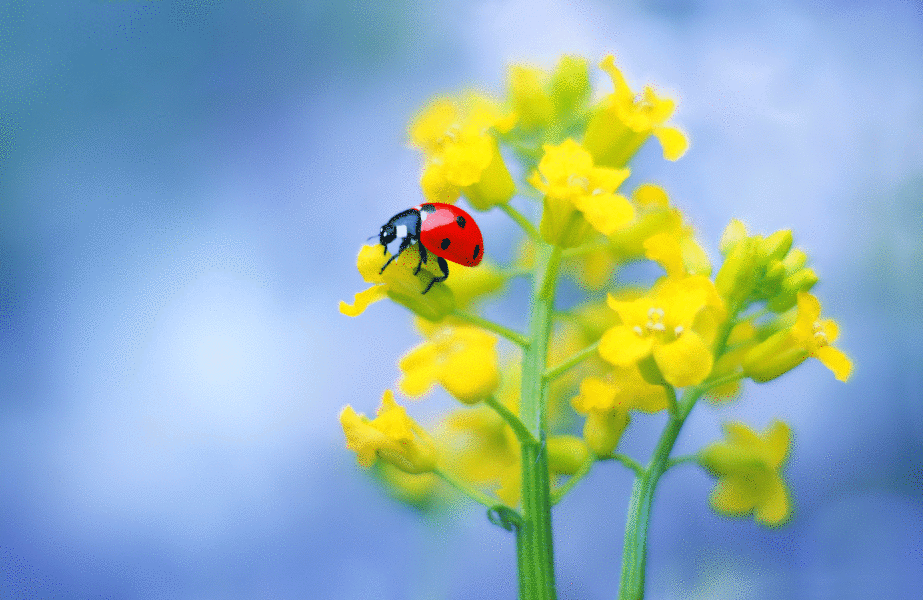# Quantify colour by {magick}

colour
r
magick
Author

Matt Dray

Published

November 25, 2018

## tl;dr

I used the {magick} package in R to map an image’s colours to their nearest match from a simplified palette, then quantified how much of the image was covered by each colour in that palette.

Note

I later learnt about {colorfindr} by David Zumbach, which can extract colours from images, provide composition details and generate palettes. Check it out.

## Art of the possible

What might be a relatively simple and straightforward way to do this in R? By ‘simple’ I mean we don’t want to do any hard work. We don’t want to consider any colour theory1 and we want to stick to simple, easily-named colours like ‘green’.2

So, we can do the following:

2. Prepare a set of ‘simple colours’
3. Map the simple colours to the image
4. Quantify the colours

## It’s a kind of ImageMagick

The {magick} R package is an implementation of ImageMagick, an open-source software suite whose emphasis is image manipulation from the command line. The flexibility of {magick} can be seen in its vignette.

The package was created and is maintained by Jeroen Ooms, a software engineer and postdoc at rOpenSci, a collective that seeks to develop tools for open and reproducible research.

rOpenSci hosted a workshop from Ooms about working with images in R and the presentation slides caught my attention. I’ve used some of Jeroen’s code below.

## Code

First we need to load our packages; all are available from CRAN.

``````suppressPackageStartupMessages({
library(dplyr)
library(tibble)
library(magick)
})``````

I’ve chosen a colourful image to use for our test case: it’s a picture of a bunch of Lego Duplo bricks.3

We’ll use `image_read()` to read the JPEG as an object of class ‘magick’ and then `image_scale()` to reduce the image size and save some space.

Printing the image also gives us some details of format, dimensions, etc.

``````# Path to the image

# Read as magick object and resize
image_scale(geometry = c("x600"))

print(duplo)``````
``````# A tibble: 1 × 7
format width height colorspace matte filesize density
<chr>  <int>  <int> <chr>      <lgl>    <int> <chr>
1 JPEG     900    600 sRGB       FALSE        0 72x72  ``````### Prepare simple colours

We’ll map a set of simple colours to the test image. This means that the colours from the test image will be replaced by the ‘closest’ colour from our simple set.

One way to do this is to define our simple colour set and create an image from them. In this case I’m taking just six colours.

``````# Generate named vector of 'simple' colours
cols_vec <- setNames(
c("#000000", "#0000ff", "#008000", "#ff0000", "#ffffff", "#ffff00"),
c("black", "blue", "green", "red", "white", "yellow")
)``````

Then we can plot squares of these colours, using `image_graph()` to read them as magick-class objects.4 My method here is not the most efficient, but you can see the output is an image that contains our six colours.

Click for code
``````# For each vector element (colour) create a square of that colour
for (i in seq_along(cols_vec)) {
fig_name <- paste0(names(cols_vec)[i], "_square")  # create object name
assign(
fig_name,  # set name
image_graph(width = 100, height = 100, res = 300)  # create magick object
)
par(mar = rep(0, 4))  # set plot margins
plot.new()  # new graphics frame
rect(0, 0, 1, 1, col = cols_vec[i], border = cols_vec[i])  # build rectangle
assign(fig_name, magick::image_crop(get(fig_name), "50x50+10+10")) # crop
dev.off()  # shut down plotting device
rm(i, fig_name)  # clear up
}

# Generate names of the coloured square objects
col_square <- paste0(names(cols_vec), "_square")

# Combine magick objects (coloured squares)
simple_cols <- image_append(c(
get(col_square), get(col_square), get(col_square),
get(col_square), get(col_square), get(col_square)
))``````
``print(simple_cols)``
``````# A tibble: 1 × 7
format width height colorspace matte filesize density
<chr>  <int>  <int> <chr>      <lgl>    <int> <chr>
1 PNG      300     50 sRGB       TRUE         0 300x300``````#### Map to the image

Now we can apply the simple colour set to the test image using `image_map()`.

``duplo_mapped <- image_map(image = duplo, map = simple_cols)``

And we can use `image_animate()` to see the difference between the two.

``image_animate(c(duplo, duplo_mapped), fps = 1)``Great. You can see where the original colours have been replaced by the ‘closest’ simple colours.

Note in particular where the more reflective surfaces are mapped to white than the actual brick colour.

This is okay: the brick may be blue, but we’ve only defined one shade of blue. If a particular shade is closer to white, then so be it.

### Quantify the colours

Now we can take this mapped image and quantify how much of the image belongs to each colour. Imagine we’ve broken the image into pixels and then we’re counting how many belng to each of our six colours.

``````# Function to count the colours (adapted from Jeroen Ooms)
count_colors <- function(image) {
data <- image_data(image) %>%
apply(2:3, paste, collapse = "") %>%
as.vector %>% table() %>%  as.data.frame() %>%
setNames(c("hex", "freq"))
data\$hex <- paste("#", data\$hex, sep="")
return(data)
}

# Dataframe of dominant colours
duplo_col_freq <- duplo_mapped %>%
count_colors() %>%
left_join(
enframe(cols_vec) %>% rename(hex = value),
by = "hex"
) %>%
arrange(desc(freq)) %>%
mutate(percent = 100*round((freq/sum(freq)), 3)) %>%
select(
`Colour name` = name, Hexadecimal = hex,
`Frequency of colour` = freq, `Percent of image` = percent
)

duplo_mapped  # see mapped image again````````knitr::kable(duplo_col_freq)  # quantify colour``
Colour name Hexadecimal Frequency of colour Percent of image
red #ff0000 132135 24.5
white #ffffff 107841 20.0
black #000000 103645 19.2
yellow #ffff00 79750 14.8
green #008000 64868 12.0
blue #0000ff 51761 9.6

So red makes up almost a quarter of the image, with white and black just behind. This makes sense: many of the bricks are red and much of the shadow areas of yellow bricks were rendered as red, while black and white make up many of the other shadows and reflective surfaces.

## And so we must p-art

So, you can map a simple colour set to an image with {magick} and then quantify how much of the image is covered by that simple set.

Of course, there are many possibilities beyond what’s been achieved here. For example, you could create a tool where the user chooses a colour and images are returned in order of dominance for that colour. You could also write this all into a function that takes a folder of images and returns the percentage of each colour in each image.

Below are some additional examples of the approach taken in this post.

### Reef fish

Click for details
``````reef_path <- "https://upload.wikimedia.org/wikipedia/commons/0/05/100%25_reef-fish_Endemism_at_90_m_off_Kure_Atoll.jpg"

image_scale(geometry = c("x600"))

reef_mapped <- image_map(image = reef, map = simple_cols)

reef_col_freq <- reef_mapped %>%
count_colors() %>%
left_join(
enframe(cols_vec) %>% rename(hex = value),
by = "hex"
) %>%
arrange(desc(freq)) %>%
mutate(percent = 100*round((freq/sum(freq)), 3)) %>%
select(
`Colour name` = name, Hexadecimal = hex,
`Frequency of colour` = freq, `Percent of image` = percent
)

reef_animate <- image_animate(c(reef, reef_mapped), fps = 1)``````Colour name Hexadecimal Frequency of colour Percent of image
blue #0000ff 317143 49.5
black #000000 214642 33.5
green #008000 76234 11.9
yellow #ffff00 13296 2.1
red #ff0000 10086 1.6
white #ffffff 8799 1.4

### Hong Kong lights

Click for details
``````neon_path <- "https://upload.wikimedia.org/wikipedia/commons/thumb/b/b0/Neon_lights%2C_Nathan_Road%2C_Hong_Kong.jpg/900px-Neon_lights%2C_Nathan_Road%2C_Hong_Kong.jpg"

image_scale(geometry = c("x600"))

neon_mapped <- image_map(image = neon, map = simple_cols)

neon_col_freq <- neon_mapped %>%
count_colors() %>%
left_join(
enframe(cols_vec) %>% rename(hex = value),
by = "hex"
) %>%
arrange(desc(freq)) %>%
mutate(percent = 100*round((freq/sum(freq)), 3)) %>%
select(
`Colour name` = name, Hexadecimal = hex,
`Frequency of colour` = freq, `Percent of image` = percent
)

neon_animate <- image_animate(c(neon, neon_mapped), fps = 1)``````Colour name Hexadecimal Frequency of colour Percent of image
black #000000 191573 71.0
green #008000 23126 8.6
blue #0000ff 18454 6.8
red #ff0000 17553 6.5
yellow #ffff00 10872 4.0
white #ffffff 8422 3.1

Click for details
``````lbird_path <- "https://upload.wikimedia.org/wikipedia/commons/d/d5/Erysimum_Cheiranthoides_%28215134987%29.jpeg"

image_scale(geometry = c("x600"))

lbird_mapped <- image_map(image = lbird, map = simple_cols)

lbird_col_freq <- lbird_mapped %>%
count_colors() %>%
left_join(
enframe(cols_vec) %>% rename(hex = value),
by = "hex"
) %>%
arrange(desc(freq)) %>%
mutate(percent = 100*round((freq/sum(freq)), 3)) %>%
select(
`Colour name` = name, Hexadecimal = hex,
`Frequency of colour` = freq, `Percent of image` = percent
)

lbird_animate <- image_animate(c(lbird, lbird_mapped), fps = 1)``````Colour name Hexadecimal Frequency of colour Percent of image
white #ffffff 302626 54.6
blue #0000ff 118010 21.3
yellow #ffff00 101797 18.4
green #008000 27892 5.0
red #ff0000 2322 0.4
black #000000 1153 0.2

## Environment

Session info
``Last rendered: 2023-08-05 17:29:58 BST``
``````R version 4.3.1 (2023-06-16)
Platform: aarch64-apple-darwin20 (64-bit)
Running under: macOS Ventura 13.2.1

Matrix products: default
BLAS:   /Library/Frameworks/R.framework/Versions/4.3-arm64/Resources/lib/libRblas.0.dylib
LAPACK: /Library/Frameworks/R.framework/Versions/4.3-arm64/Resources/lib/libRlapack.dylib;  LAPACK version 3.11.0

locale:
 en_US.UTF-8/en_US.UTF-8/en_US.UTF-8/C/en_US.UTF-8/en_US.UTF-8

time zone: Europe/London
tzcode source: internal

attached base packages:
 stats     graphics  grDevices utils     datasets  methods   base

other attached packages:
 magick_2.7.4 tibble_3.2.1 dplyr_1.1.2

loaded via a namespace (and not attached):
 vctrs_0.6.3       cli_3.6.1         knitr_1.43.1      rlang_1.1.1
 xfun_0.39         png_0.1-8         generics_0.1.3    jsonlite_1.8.7
 glue_1.6.2        htmltools_0.5.5   fansi_1.0.4       rmarkdown_2.23
 evaluate_0.21     fontawesome_0.5.1 fastmap_1.1.1     yaml_2.3.7
 lifecycle_1.0.3   compiler_4.3.1    htmlwidgets_1.6.2 Rcpp_1.0.11
 pkgconfig_2.0.3   rstudioapi_0.15.0 digest_0.6.33     R6_2.5.1
 tidyselect_1.2.0  utf8_1.2.3        curl_5.0.1        pillar_1.9.0
 magrittr_2.0.3    withr_2.5.0       tools_4.3.1      ``````

## Footnotes

1. Just as well, because I’m colourblind.↩︎

2. There are five named versions of olive drab in R’s named palette.↩︎

3. Photo by Arto Alanenpää, CC0-BY-4.0 from Wikimedia Creative Commons.↩︎

4. Artefacts introduced during compression of PNGs and JPGs might mean that your set of six colours ends up being more than six. It’s preferable to generate our colour set within R, inside `image_graph()`, so that we have only our six defined colours.↩︎

CC BY-NC-SA 4.0